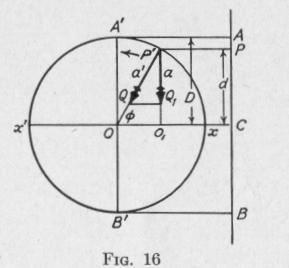Previous Page Index(For best results view this page as a PDF) Next Page

22   PRINCIPLES OF ELEMENTARY DYNAMICS

fixed in the body at which the weight of the body is applied. Thus, it is assumed that every body has a center of gravity coincident with the point at which the resultant of the gravitational forces acting on the body would be applied if they were parallel.

If a body or system of bodies be conceived to be divided into particles of equal mass, then that point, the distance of which from any given plane is equal to the average distance from that plane of all the constituent particles, is termed the center of mass, or center of inertia, of the body or system of bodies.

If we represent by m,, m2, etc., the masses of the particles composing a body of total mass M, and by x,, x2, etc., the respective distances of these particles from any given plane, then it can be shown that the distance of the center of mass of the body from the

given plane is   X = m,xl + m2x2 + etc.   29 M

()

The center of mass has the following properties:

(a) The center of mass is coincident with the centroid.

(b) The weight of a body acts approximately at its center of mass.

(c) If the resultant of all the forces acting on a rigid body is a single force the line of action of which passes through the center of mass of the body, the motion of the body is without angular acceleration.

(d) The center of mass of a body is so situated that the linear motion of the body will not be changed if the total mass were concentrated at this point and all the forces acting on the body were transferred to this point without change of magnitude or direction.

(e) The motion of the center of mass of any material system is not affected by the internal forces between the parts of the system, but only by external forces.

(f) No material system can of itself, without the action of external forces, change the motion of its center of mass.

(g) An unconstrained body, when acted upon by a system of forces equivalent to a couple, will rotate about its center of mass with constant angular acceleration.

§2. Simple Harmonic Motion

18. Simple Harmonic Motion of Translation. - When a body moves with a reciprocating motion which has at every instant a linear acceleration directed toward the center of the path and that

SIMPLE HARMONIC MOTION   23

varies directly with the distance of the moving body from that point, the body is said to have a simple harmonic motion of translation.

The defining equation is

where c is a positive constant. The negative sign indicates that displacement d is measured from the equilibrium position whereas acceleration a is directed toward the equilibrium position.

Any elastic body that is distorted within the limit for which Hooke's Law is true, and then released, will thereafter vibrate with simple harmonic motion of translation. The motions of many other bodies are resultants of simple harmonic motions.

The time which elapses between two consecutive passages of the oscillating body in the same direction through any given point of its path is called the period of the motion. The maximum distance attained by the oscillating body from its position of equilibrium is called the amplitude of the motion.

It will now be shown that if a point moves with uniform speed in the circumference of a circle, the projection of the point on any straight line in the plane of the circle moves with simple harmonic motion of translation. This fact is the basis of our most easy methods for finding values of the period of a simple harmonic motion of translation as well as values for the displacement, acceleration and velocity of a body vibrating with simple harmonic motion.

Let a particle P, Fig. 16, move with uniform speed in the circumference of a circle P'A'B', of radius r, and let P be the pro

jection of this point on any right line A B in the plane of the circle. As P moves with uniform speed in the circumference of the circle, its projection P oscillates back and forth through a middle position C between two extreme positions A and B. The sort of motion described by P along the line A B will now be investigated.

As the particle P moves with uni

form speed in the circumference of a

circle there is a constant acceleration

directed toward the center of the circle. In Fig. 16, this radial acceleration a' is represented by the line P'Q. Let the compo-Previous Page Index(For best results view this page as a PDF) Next Page